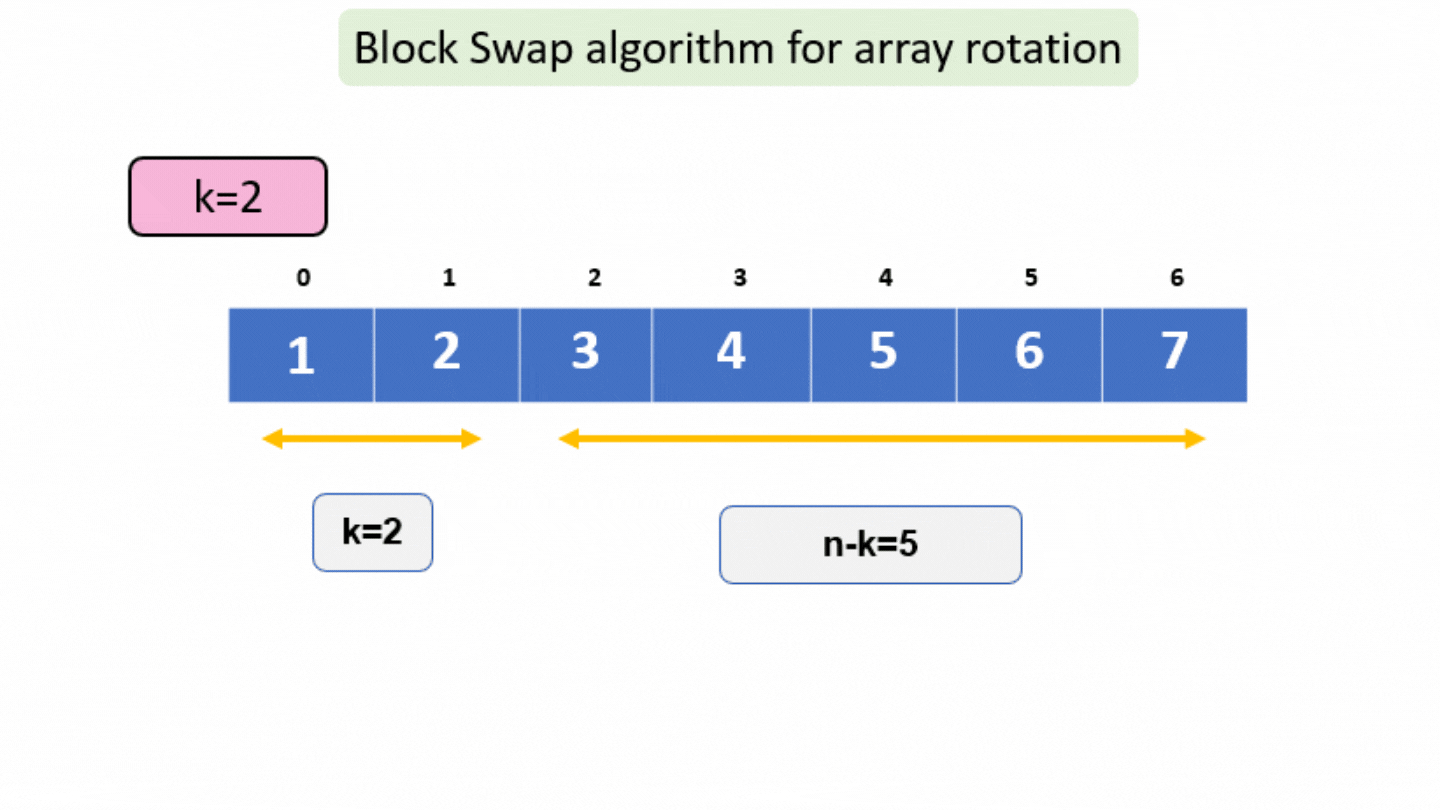# Rotate array by K elements : Block Swap Algorithm

In this article we will learn a very popular algorithm for “Rotate array by K elements : Block Swap Algorithm”.

Problem Statement: Given an array of n size, rotate the array by k elements using the Block Swap Algorithm.

Examples:

```Example 1:
Input: N = 5, array[] = {1,2,3,4,5} K=2
Output: {3,4,5,1,2}
Explanation: Rotate the array to right by 2 elements.

Example 2:
Input: N = 7, array[] = {1,2,3,4,5,6,7} K=3
Output: {4,5,6,7,1,2,3}
Explanation: Rotate the array to right by 3 elements.```

### Solution

Disclaimer: Don’t jump directly to the solution, try it out yourself first.

Let us first understand what is block swap algorithm

Divide the given array into two subarrays A and B where A stores the first ‘k’ elements and
B store next ‘n-k’ elements. If the size of both subarrays is not equal then perform block swap b/w A and B, where the block size is the size of smaller subarray and then reduce the size of subarray by block size. Repeat this until the size of both subarrays become equal.

Solution 1:

Approach

For given k,

Two cases can arise in an array:-

Case 1: when k<n-k

For this, we need to swap the first k elements with the last k elements in the given part of the array.

By this last k elements come to their correct position.

Case 2: when k>n-k

For this, we need to swap the last n-k elements with the first n-k elements in the given part of the array.

By this first n-k elements come to their correct position.

Case 3: when k=n-k

For this, just swap the first half and the second part of the given array.

And just return

For eg,

arr[]={1,2,3,4,5,6,7} , n=7 , k=2Code:

## C++ Code

``````#include <iostream>
using namespace std;
void swap(int arr[], int a, int b, int k)
{
for (int i = 0; i < k; i++)
{
int temp = arr[a + i];
arr[a + i] = arr[b + i];
arr[b + i] = temp;
}
}
void BlockSwap(int arr[], int k, int n)
{
if (k == 0 || k == n)
return;
// If first part and second part are of same size
if (k == n - k)
{
swap(arr, 0, n - k, k);
return;
}
// If first part of array is of smaller size
else if (k < n - k)
{
swap(arr, 0, n - k, k);
BlockSwap(arr, k, n - k); // second part of array is taken now
}
// If second part of array is of smaller size
else
{
swap(arr, 0, k, n - k);
BlockSwap(arr + n - k, 2 * k - n, k);
}
}
int main()
{
int arr[] = {1, 2, 3, 4, 5, 6, 7};
int n = 7;
int k = 2;
BlockSwap(arr, k, n);
cout << "After Rotating the array " << endl;
for (int i = 0; i < n; i++)
cout << arr[i] << " ";
return 0;
}
``````

Output:

After Rotating the array
3 4 5 6 7 1 2

Time Complexity: O(n)

Space Complexity: O(1)

## Java Code

``````import java.util.*;
public class Main {
public static void swap(int[] arr, int a, int b, int k) {
for (int i = 0; i < k; i++) {
int temp = arr[a + i];
arr[a + i] = arr[b + i];
arr[b + i] = temp;
}
}
public static void BlockSwap(int[] arr, int i, int k, int n) {
if (k == 0 || k == n)
return;
// If first part and second part are of same size
if (k == n - k) {
swap(arr, i, n - k + i, k);
return;
}
// If first part of array is of smaller size
else if (k < n - k) {
swap(arr, i, n - k + i, k);
BlockSwap(arr, i, k, n - k); // second part of array is taken now
}
// If second part of array is of smaller size
else {
swap(arr, i, k, n - k);
BlockSwap(arr, n - k + i, 2 * k - n, k);
}
}
public static void main(String args[]) {
int[] arr = {1,2,3,4,5,6,7};
int n = 7;
int k = 2;
BlockSwap(arr, 0, k, n);
System.out.println("After Rotating the array ");
for (int i = 0; i < n; i++)
System.out.print(arr[i] + " ");
}
}
``````

Output:

After Rotating the array
3 4 5 6 7 1 2

Time Complexity: O(n)

Space Complexity: O(1)

Special thanks to Gurmeet Singh for contributing to this article on takeUforward. If you also wish to share your knowledge with the takeUforward fam, please check out this article